# AP Physics C: Mechanics Practice Test 2

### Test Information10 questions13 minutes

1. A satellite is in circular orbit around Earth. If the work required to lift the satellite to its orbit height is equal to the satellite's kinetic energy while in this orbit, how high above the surface of Earth (radius = R) is the satellite?

2.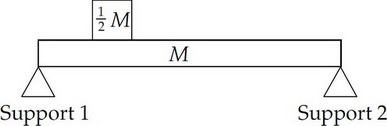The figure above shows a uniform bar of mass M resting on two supports. A block of mass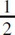M is placed on the bar twice as far from Support 2 as from Support 1. If F1 and F2 denote the downward forces on Support 1 and Support 2, respectively, what is the value of F2/F1?

3. A rubber ball (mass = 0.08 kg) is dropped from a height of 3.2 m, and after bouncing off the floor, rises almost to its original height. If the impact time with the floor is measured to be 0.04 s, what average force did the floor exert on the ball?

4. A disk of radius 0.1 m initially at rest undergoes an angular acceleration of 2.0 rad/s2. If the disk only rotates, find the total distance traveled by a point on the rim of the disk in 4.0 s.

5.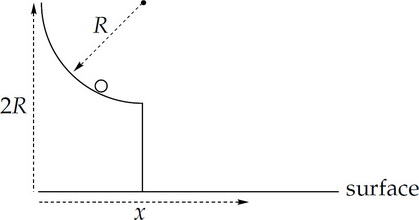In the figure above, a small ball slides down a frictionless quarter-circular slide of radius R. If the ball starts from rest at a height equal to 2R above a horizontal surface, find its horizontal displacement, x, at the moment it strikes the surface.

6.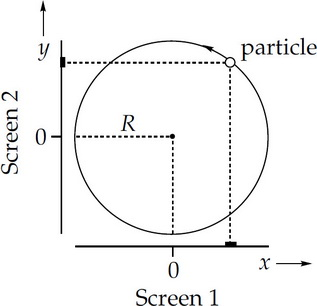The figure above shows a particle executing uniform circular motion in a circle of radius R. Light sources (not shown) cause shadows of the particle to be projected onto two mutually perpendicular screens. The positive directions for x and y along the screens are denoted by the arrows. When the shadow on Screen 1 is at position x = –(0.5)R and moving in the +x direction, what is true about the position and velocity of the shadow on Screen 2 at that same instant?

7.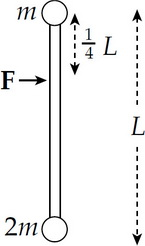The figure shows a view from above of two objects attached to the end of a rigid massless rod at rest on a frictionless table. When a force F is applied as shown, the resulting rotational acceleration of the rod about its center of mass is kF/(mL). What is k?

8. A lightweight toy car crashes head-on into a heavier toy truck. Which of the following statements is true as a result of the collision?

I. The car will experience a greater impulse than the truck.

II. The car will experience a greater change in momentum than the truck.

III. The magnitude of the acceleration experienced by the car will be greater than that experienced by the truck.

9. A homogeneous bar is lying on a flat table. Besides the gravitational and normal forces (which cancel), the bar is acted upon by exactly two other external forces, F1 and F2, which are parallel to the surface of the table. If the net force on the rod is zero, which one of the following is also true?

10. An astronaut lands on a planet whose mass and radius are each twice that of Earth. If the astronaut weighs 800 N on Earth, how much will he weigh on this planet?